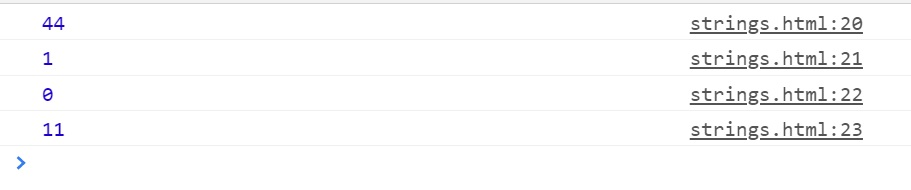# JavaScript String Methods

JavaScript String Methods

With the help of string methods, we can simply do many different things with the strings(this is basically doing some operations on the strings, like calculating the length of the string, converting the string to lowercase or uppercase, and so on…). Let’s have a look at some different methods related to the strings here –

#### Calculating the length of a string (length property)

The first thing that we are talking about here, is not actually a method, but a property, using which, we are calculating the length of the string.

So, if you want to get the length of a string, it’s super easy. You just need to use the length property. Following is an example that demonstrates the use of the length property to calculate the length of a string. The length of a string is nothing but the number of characters in the string. A string with no characters has a length of zero, and a character is just a single-length string –As you can see, we have used the property length here, and notice how are we using it, like the variable name, followed by the dot, and then the length property. Have a look at the output now →As you can see, we could get the length of the different strings that we have made in the program. If you ever need the length of some string, we can make use of the length property, and easily get the length of the string.